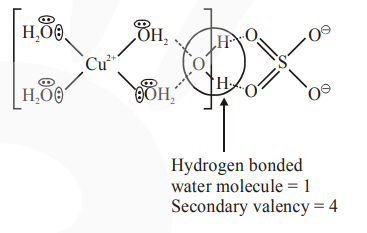# The secondary valency and the number of hydrogen bonded`
Question:

The secondary valency and the number of hydrogen bonded water molecule(s) in $\mathrm{CuSO}_{4} \cdot 5 \mathrm{H}_{2} \mathrm{O}$, respectively, are :

1. 6 and 4

2. 4 and 1

3. 6 and 5

4. 5 and 1

Correct Option: , 2

Solution: